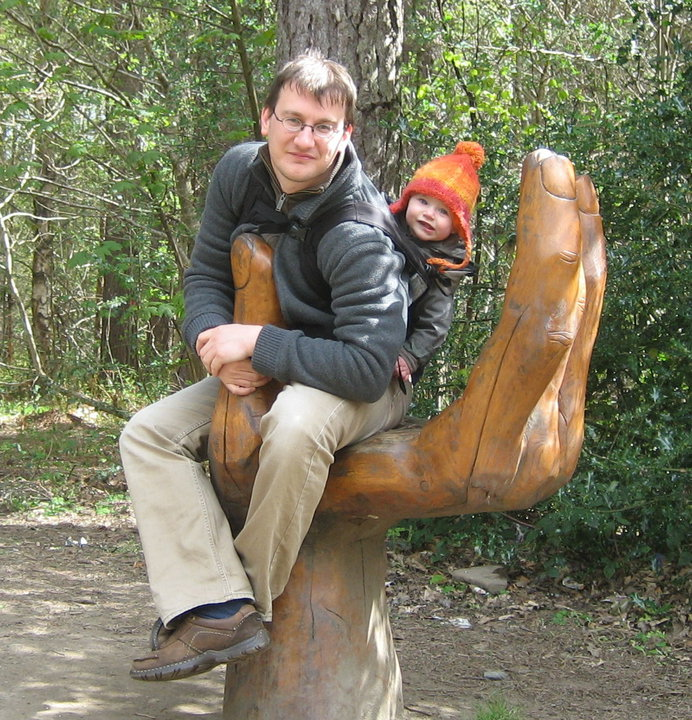I work at the Korteweg-de Vries institute of mathematics at the University of Amsterdam. I teach Algebra I in the Bachelor Mathematics, Mirror symmetry in the Master Mathematical Physics and a course on chaos theory in the Bachelor Beta-Gamma.

## Research interests

•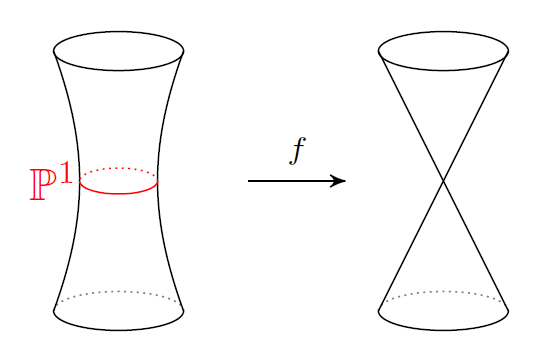Resolutions of singularities:
Noncommutative symplectic geometry, formally smooth algebras, derived equivalences between noncommutative algebras and algebraic varieties.
•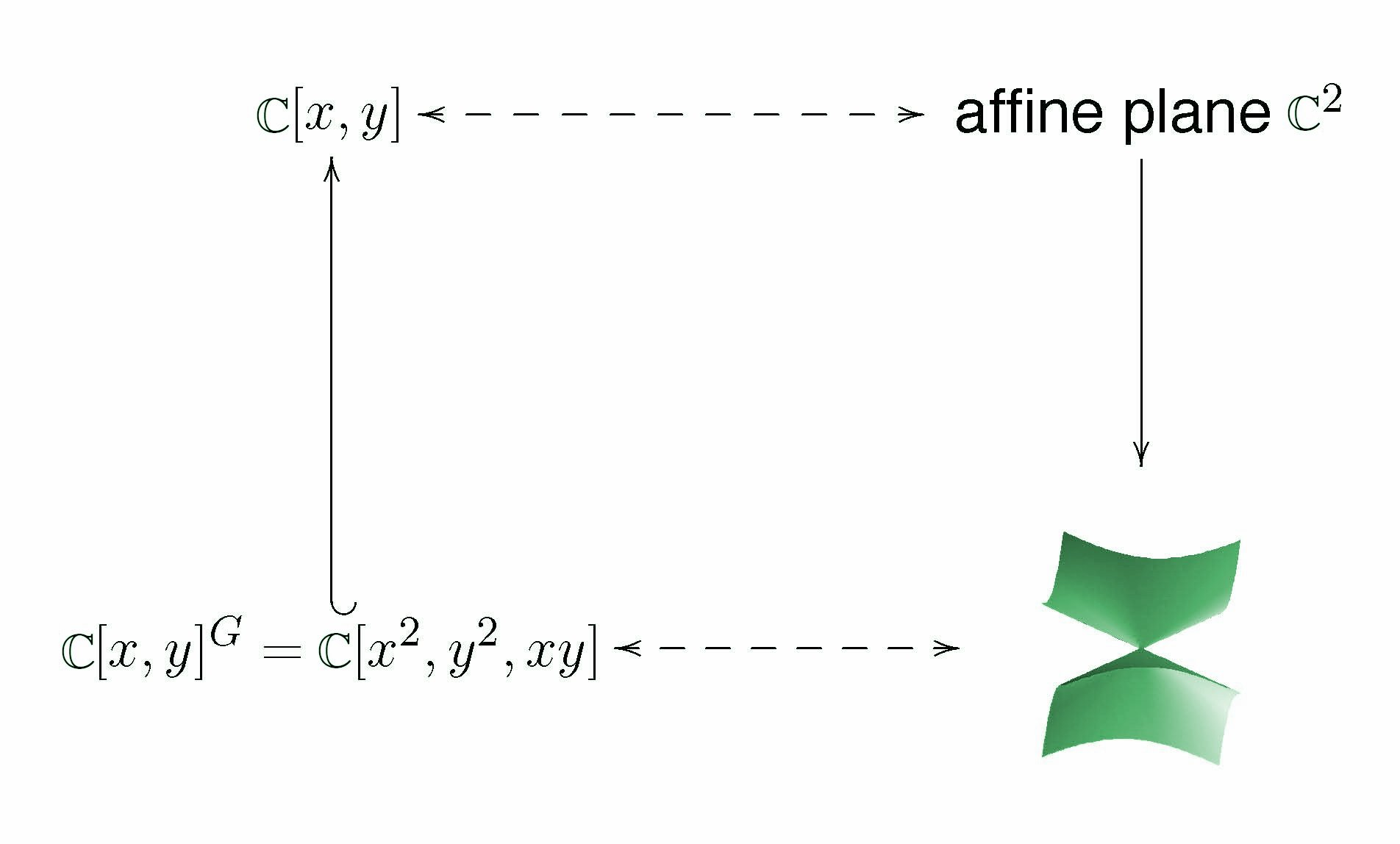Geometric invariant theory:
quotient singularities, moduli spaces of stable representations, slice theorems. Stability conditions.
•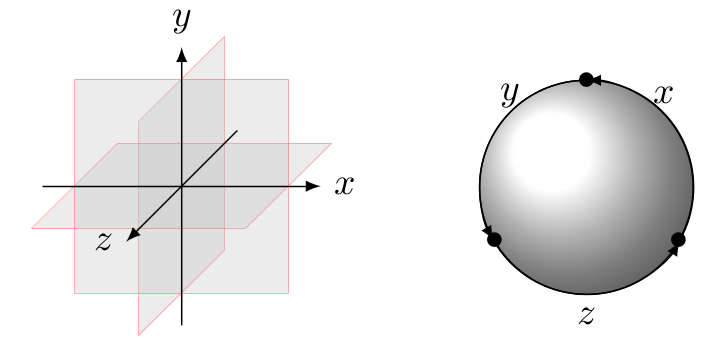Homological mirror symmetry:
Matrix factorizations for noncommutative algebras, Fukaya categories of (punctured) surfaces.
•Dimer models:
Representations of Jacobi Algebras, Noncomutative crepant resolutions and the relation to mirror symmetry
•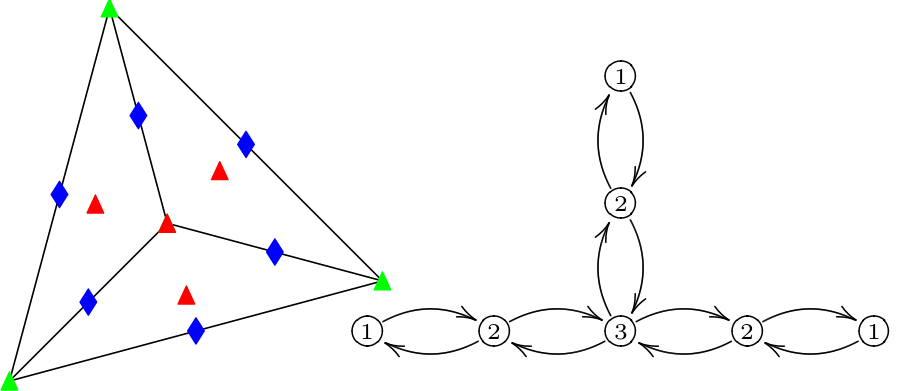Representation theory of quivers:
Local quivers, quivers with potentials, preprojective algebras, Mckay correspondence. Applications of quivers in theoretical physics and topology.